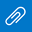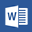# Year 4: Home learning update for 8/6

Good morning!

Today I’d like you to multiply three-digit numbers by one-digit numbers (e.g. 318 x 4).

The great thing is, like the subtractions sums, there are lots of ways you can do this!
Method 1 – Breaking it down using place value.
300 x 4 = 1200 (If you didn’t know this do 3 x 4 = 12 first to help you)
10 x 4 = 40
8 x 4 = 16
Other ways to work out the answer include multiplying the 3-digit number by a smaller number, e.g. 2, and doubling the answer. You could also use a column method to work out the answer or the grid method (videos to watch on Google Classroom).Lesson-Powerpoint.pptGood-and-Bad-Football-exposition.docTable-Marking-Texts-against-SC.docx